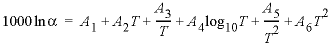| Next || Previous || Top |

## NAMED_EXPRESSIONS

This keyword data block is used to assign names to expressions for equilibrium constants and fractionation factors. The named expressions are useful to simplify definition of thermodynamic data for isotopic species in SOLUTION_SPECIES and PHASES data blocks, and are used in the ISOTOPE_ALPHAS data block to provide printing of fractionation factors to the output file. The NAMED_EXPRESSIONS data block is used in the database file iso.dat and llnl.dat databases.

###### Example data block
`Line 0:  NAMED_EXPRESSIONS`
`Line 1:  Log_alpha_13C_CO2(aq)/CO2(g)             `
`Line 2:    -ln_alpha1000			-0.9	0.0	0.0	0.0	.0063e6`
`Line 1a: Log_alpha_13C_HCO3-/CO2(aq) `
`Line 3:    -add_logk			Log_alpha_13C_HCO3-/CO2(g)				1`
`Line 3a:   -add_logk			Log_alpha_13C_CO2(aq)/CO2(g)				-1`
###### Explanation

Line 0: NAMED_EXPRESSIONS

NAMED_EXPRESSIONS is the keyword for the data block. No other data are input on the keyword line. Optionally, NAMED_LOG_K , NAMED_EXPRESSIONS , or NAMED_ANALYTICAL_EXPRESSIONS .

Line 1: Name

Name --Name for the expression.

Line 2: -ln_alpha1000 A 1 , A 2 , A 3 , A 4 , A 5 , A 6

-ln_alpha1000 --An analytical expression for a fractionation factor is defined. The six coefficients are applied to the expression, where T is temperature in kelvin. If less than six parameters are defined, the undefined parameters are assumed to be zero. This identifier may be used only once in the definition of a named expression. Optionally, ln_alpha1000 or -ln [ _alpha1000 ].

A 1 , A 2 , A 3 , A 4 , A 5 , A 6 --Coefficients for the analytical expression for a fractionation factor.

-add_logk --The named_expression is used in calculating the value for Name from the preceding Line 1. This identifier may be used multiple times in the definition of a named expression. Optionally, add_logk , add_log_k , -ad [ d_logk ] or -ad [ d_log_k ].

named_expression --Name of an expression defined in a NAMED_EXPRESSIONS data block.

coefficient --The coefficient is multiplied by the value of the named_expression when calculating the value of Name .

###### Notes

The NAMED_EXPRESSIONS data block is implemented to avoid multiple definitions of analytical expressions, to simplify combining expressions for fractionation factors, and to preserve relationships among isotopic fractionation factors and equilibrium constants. It is used to implement the individual isotope equilibrium constant approach of Thorstenson and Parkhurst (2000, 2004).

Fractionation factors are commonly reported with analytical expressions for 1000ln(α). The -ln_alpha1000 identifier allows definition of a fractionation factor in this form. However, the analytical expression is immediately converted to an expression for the log base 10 fractionation factor. All combinations of named expressions are performed with log base 10 operations.

The -add_logk identifier is used to sum up multiple expressions to produce a new expression. In the Example data block, the fractionation factor between HCO 3 - and CO 2(aq) is defined as a combination of the fractionation factor between HCO 3 - and CO 2(g) and the fractionation factor between CO 2(g) and CO 2(aq) . It is good practice to define the analytical expressions for fractionation factors only once, and then use the -add_logk identifier to add it to form other expressions. In this way, the relationships among fractionation factors are preserved, even if the analytical expression is changed.

The database iso.dat uses the NAMED_EXPRESSIONS data block to implement isotope fractionation factors. Within that data block, the only identifiers used are -ln_alpha1000 and -add_logk . However, for completeness, it is also possible to use the identifiers -analytical_expression , -log_k , and -delta_h to define named expressions. Descriptions of these identifiers can be found in the PHASES and SOLUTION_SPECIES data blocks.

Named expressions are used to define the equilibrium constants for isotopic species in the SOLUTION_SPECIES and PHASES data blocks of the iso.dat database. Named expressions are used in the ISOTOPE_ALPHAS data block to print values of analytical expressions to the output file. The values of the named expressions for the current temperature can be obtained with the Basic function LK_NAMED(“ name ”).

###### Example problems

The NAMED_EXPRESSIONS data block is used in the iso.dat and llnl.dat databases.

###### Related keywords

| Next || Previous || Top |